Relation Between Yarn Count and Diameter|textile study center
Home Fabric Engineering Relation Between Yarn Count and Diameter & count calculation for ply yarn

# Relation Between Yarn Count and Diameter & count calculation for ply yarn

6.20K
0#### Md Sohanur Rahman Sobuj

Co-founder , Admin & Author at Textile Study Center
Student of Bangladesh University of Textiles (BUTEX) Department: Apparel Engineering#### +Md Sohanur Rahman Sobuj## Relation Between Yarn Count and Diameter & count calculation for ply yarn :

Count calculation for ply yarn :

We can make a plied yarn by twisting two single yarns together. The resultant yarn count is calculated as follows:

Expression of Resultant Count:

Resultant count of indirect system= Number of plies/Count of single yarn Or Count of single yarn/Number of plies

Resultant count of direct system= Count of single yarn × Number of plies Or Resultant count(count of ply or 3,4….yarn)/Number of plies

For example,

2/24s cotton count: This indicates a yarn made from two 24s cotton count yarns twisted together.

80 Tex x 2: This indicates a yarn made from twisting together two 80 Tex yarns. This type of designation is generally used with woolen yarns.

R 74 Tex/2: This indicates a yarn made from twisting two yarns together whose resultant count is 74 Tex. This type of designation is generally used with worsted yarns.

Math: A two ply yarn in Tex is composed of one thread 40 Tex, one thread unknown count and has a resultant count of 100 Tex. What is the count of the other component yarn?

Soln: We know that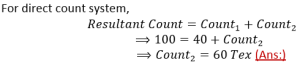Math: A resultant two ply yarn count of 16 worsted has one component yarn of 36 worsted. What is the count of the other component?

Soln: We know that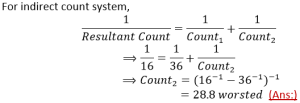Math: A three ply yarn is composed of one thread of 56 worsted, one thread of 48 worsted and one thread of 2/80 cotton. What is the count of resultant yarn?

Soln: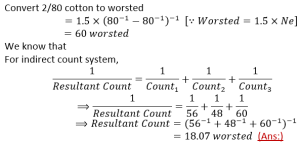Count calculation for ply yarn with contraction:

Normally, when two single yarns are twisted together one should expect some contraction or some increase in length depending on the twisting direction. A contraction will result in a yarn slightly coarser than the estimated value. In order to correct for this difference, typically 5% to 10% contraction or extension should be accounted for.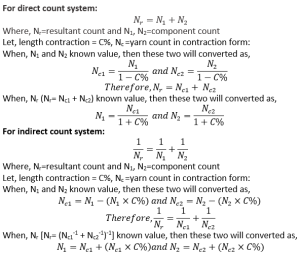Math: Suppose two yarns of 24/1 Nm are going to be plied with 4% contraction. Find its resultant count in metric system.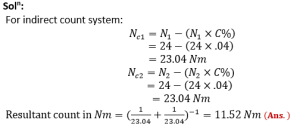Math: Suppose you are provided a cotton yarn of 1500 km of 2/24s found at completely dry condition. Calculate the resultant count in Tex at standard atmospheric condition with 7% contraction.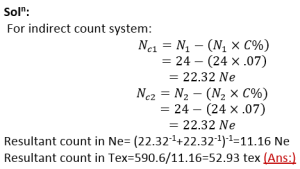Relation Between Yarn Count and Diameter :

Before considering cloth setting formulae it is important to fully understand the relationship between the count of a yarn and its diameter (or thickness). If the diameter is increased or decreased then the yarn count is changed.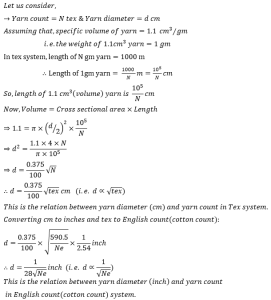(6203)#### Md Sohanur Rahman Sobuj

Co-founder , Admin & Author at Textile Study Center
Student of Bangladesh University of Textiles (BUTEX) Department: Apparel Engineering#### +Md Sohanur Rahman Sobuj